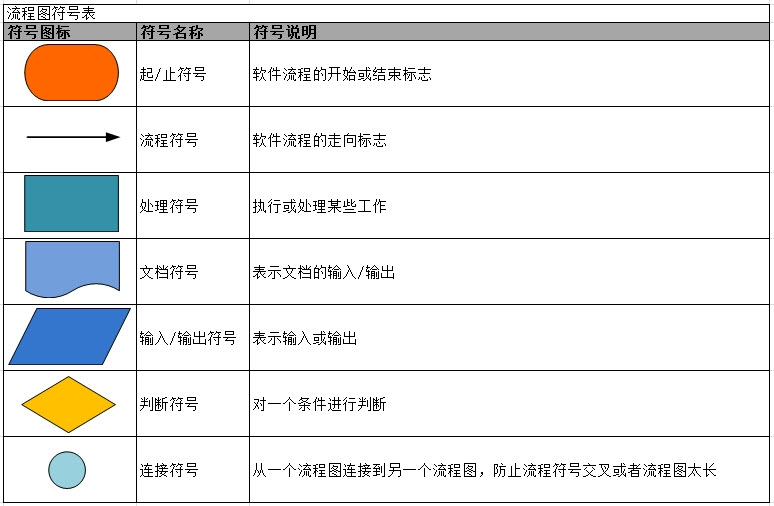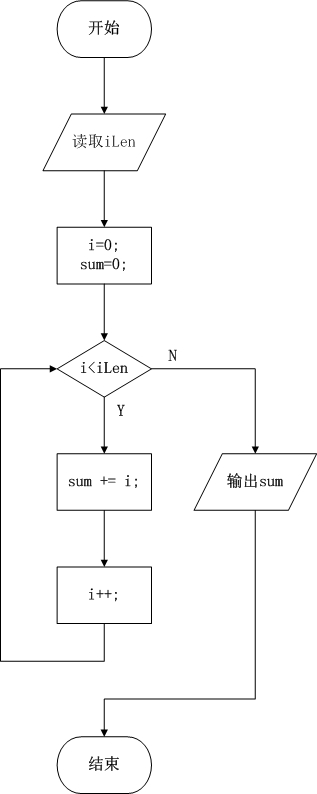# 流程图介绍## 流程图示例

``````import java.io.BufferedReader;
import java.io.IOException;

public class MathDemo {

public static void main(String[] args) {

// 计算"0"到"iLen"的合
int sum = 0;
int iLen = getLen();
for (int i=0; i<iLen; i++) {
sum += i;
}
System.out.println("summary(0~"+iLen+")="+sum);
}

// 读取一个长度，默认返回100
private static int getLen() {
try {

// 读取一行，字符串
// 将该字符串转换为int整型数
int len = Integer.parseInt(str);

in.close();
return len;
} catch(IOException e) {
e.printStackTrace();
return 100;
}
}
}
``````

``````please insert a number:10
summary(0~10)=45
``````

MathDemo的作用是，让用户输入一个整数。然后计算0到"这个整数"之间的和。